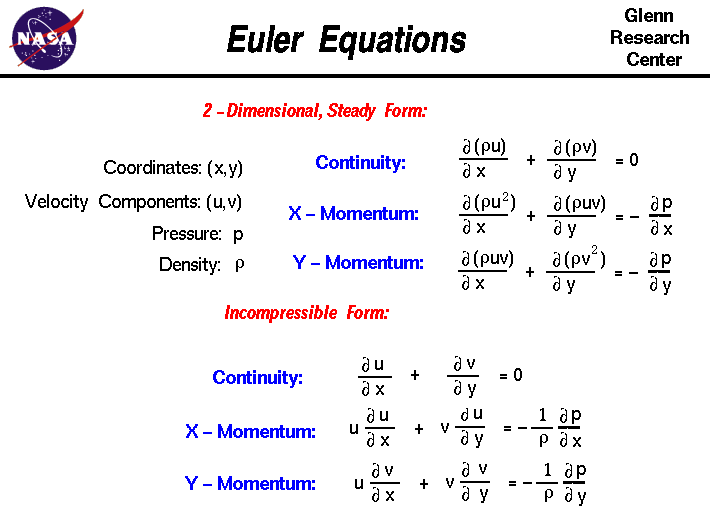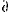+ Text Only Site
+ Non-Flash Version
+ Contact GlennOn this slide we have two versions of the Euler Equations which describe how the velocity, pressure and density of a moving fluid are related. The equations are named in honor of Leonard Euler, who was a student with Daniel Bernoulli, and studied various fluid dynamics problems in the mid-1700's. The equations are a set of coupled differential equations and they can be solved for a given flow problem by using methods from calculus. Though the equations appear to be very complex, they are actually simplifications of the more general Navier-Stokes equations of fluid dynamics. The Euler equations neglect the effects of the viscosity of the fluid which are included in the Navier-Stokes equations. A solution of the Euler equations is therefore only an approximation to a real fluids problem. For some problems, like the lift of a thin airfoil at low angle of attack, a solution of the Euler equations provides a good model of reality. For other problems, like the growth of the boundary layer on a flat plate, the Euler equations do not properly model the problem. Our world has three spatial dimensions (up-down, left-right, fore-aft) and one time dimension. In general, the Euler equations have a time-dependent continuity equation for conservation of mass and three time-dependent conservation of momentum equations. At the top of the figure, we show a simplified, two-dimensional, steady form of the Euler equations. There are two independent variables in the problem, the x and y coordinates of some domain. There are four dependent variables, the pressure p, density r, and two components of the velocity vector; the u component is in the x direction, and the v component is in the y direction. All of the dependent variables are functions of both x and y. The differential equations are therefore partial differential equations and not ordinary differential equations that you study in a beginning calculus class. You will notice that the differential symbol is different than the usual "d /dt" or "d /dx" that you see for ordinary differential equations. The symbol "" is used to denote partial differentiation. The symbol indicates that we are to hold all of the independent variables fixed, except the variable next to symbol, when computing a derivative. The set of equations are: Continuity:(r * u)/x +(r * v)/y = 0 X - Momentum:(r * u^2)/x +(r * u * v)/y = -p/x Y - Momentum:(r * u * v)/x +(r * v^2)/y = -p/y Although these equations appear very complex, undergraduate engineering students are taught how to derive them in a process very similar to the derivation that we present on the conservation of momentum web page. The two momentum equations are two-dimensional generalizations of the conservation of momentum equation. The mass flow rate equation developed on the conservation of mass web page is a one dimensional solution of the continuity equation shown here. Generalized solutions of these equations are difficult to obtain. Notice that all of the dependent variables appear in each equation. To solve a flow problem, you have to solve all three equations simultaneously; that is why we call this a coupled system of equations. There is actually another equation that is required to solve this system, since we only show three equations for four unknowns. An equation of state relates the pressure and the density of a gas. In the past, engineers made further approximations and simplifications to the equation set until they had a group of equations that they could solve. Recently, high speed computers have been used to solve approximations to the equations using a variety of techniques like finite difference, finite volume, finite element, and spectral methods. This area of study is called Computational Fluid Dynamics or CFD. One of the simplification methods used in the past was to assume that the gas was very low speed and to neglect the effects of compressibility. In an incompressible flow, the density is constant and we can remove it from the continuity equation: Continuity:u/x +v/y = 0 We can then factor the momentum equations and use the continuity equation to simplify them: X - Momentum: u *u/x + v *u/y = - [p/x] / r Y - Momentum: u *v/x + v *v/y = - [p/y] / r This set of equations was used to develop the algorithm used in the FoilSim computer program. Activities: Guided Tours Navigation ..Beginner's Guide Home Page+ Inspector General Hotline + Equal Employment Opportunity Data Posted Pursuant to the No Fear Act + Budgets, Strategic Plans and Accountability Reports + Freedom of Information Act + The President's Management Agenda + NASA Privacy Statement, Disclaimer, and Accessibility CertificationEditor: Nancy Hall NASA Official: Nancy Hall Last Updated: May 05 2015 + Contact Glenn